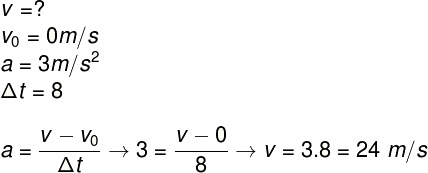Mechanics

# Acceleration

Acceleration is the physical quantity that relates the change in velocity with respect to time.

Acceleration is the physical quantity that measures the change in speed of a moving object as a function of time. The unit of acceleration in the International System of Units (SI) is m/s² (meter per second squared).

## types of acceleration

When a movement presents acceleration, its velocity changes. If the velocity of the object changes uniformly , its acceleration is constant, and its motion is uniformly varied. If the speed of the mobile changes randomly, we say that its motion is varied. Also, the movement in which there is an increase in speed is called accelerated, while the movement in which there is a decrease in speed is called retarded.

Pay attention to the sign given to acceleration: When a movement is accelerated, its acceleration will have a positive magnitude ; while in a delayed motion, the acceleration will be negative. When the motion is uniformly varied , the acceleration will always have the same magnitude.

Check out a comparative table with the different cases and types of acceleration: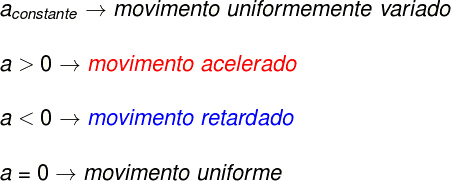Classification of different cases of acceleration.

## Average and instantaneous scalar acceleration

Velocity and acceleration are fundamentally vector quantities, as they are defined by their module , direction and sense , however, in the study of kinematics, it is common to solve problems that involve rectilinear displacements, that is, those that occur in a single direction. To solve these exercises, it is not necessary to resort to the vector formalism, therefore, exclusively in these cases, quantities such as velocity and acceleration are treated as scalar quantities.

Mean acceleration is defined as the change in speed as a function of time, while instantaneous acceleration is the measure of change in instantaneous velocity The fundamental difference between these two quantities is the time interval: the instantaneous scalar acceleration is defined for very small time intervals, close to 0: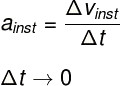The difference between average and instantaneous scalar acceleration is the time interval in which they are measured.

## acceleration formula

Acceleration can be calculated by varying the velocity with respect to time. For this, we use the following formula: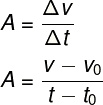a – acceleration

Δv – speed variation

Δt – time interval

v – final speed

v0 – initial speed

t – final moment

0 – initial instant

In the formula shown above, we call v and 0 the final and initial velocities of a movement, given in m/s (meters per second), while t and 0 are the initial and final moments of time .

## acceleration unit

The SI unit of acceleration is the meter per second squared (m/s²). This unit measures how many meters per second the speed of a piece of furniture changes every second.

Example:

• A car that has an acceleration of 5 m/s² has its speed increased by 5 m/s every second.
• A vehicle with an acceleration of -3 m/s² is slowed down by 3 m/s every second.

## Exercises solved

Exercise 1) A mobile has its speed changed from 36 km/h to 54 km/h, in a time interval of 2.5 s. Classify its motion and calculate the magnitude of its average acceleration.

Resolution:

As the speed of this mobile has increased, we say that its motion is accelerated. To calculate the magnitude of its acceleration, we will use the formula for the average acceleration: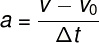The exercise only informed us the time interval in which the movement occurred, therefore, we can assume that the initial time instant (t 0 ) is equal to 0. In addition, it will be necessary to convert the velocities informed by the statement to meters per second ( m/s) dividing them by the factor 3.6. Note the calculation: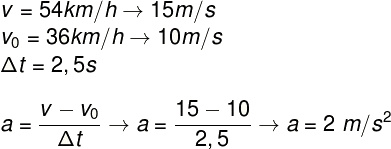Exercise 2) Upon seeing an obstacle, a driver applies the brakes and slows down at a rate of 2 m/s every second. Determine the time interval required for the vehicle to come to a complete stop, knowing that its speed at the beginning of braking was 30 m/s.

Resolution:

Analyzing the exercise statement, it can be seen that the movement is retarded and its acceleration is -2 m/s². Also, because the vehicle must come to a complete stop, its final speed is 0, and its initial speed is 30 m/s. To calculate the average acceleration, just substitute the data in the average acceleration formula: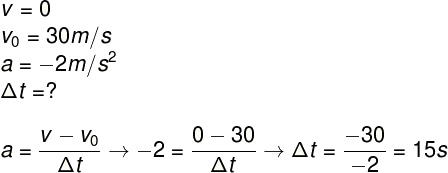Exercise 3) A mobile starts from rest and accelerates at 3 m/s² during a time interval of 8 s. Determine the velocity of this object at the end of this time interval.

Resolution:

To solve this exercise, just write down your data and substitute them in the formula for the average acceleration in order to find your speed at the end of 8 s: